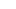# CAT Quant: Solving Circular Motion Problems

(Photo: Brkur Sigurbjrnsson)

A common question template in the Time, Speed and Distance topic of the quantitative ability section in the Common Admission Test (CAT) is based on two or three objects moving around a circular track. Let us assume that the objects have speeds ‘a’, ‘b’ and ‘c’ and are moving on a track of length ‘L’. Given below is a set of formulae that can be used for solving such questions. I have assumed that a > b > c.These formulae use the concepts of relative speed.

• Case 1 & 3 – When two bodies are moving in the same direction, their relative speed is (a-b).
• Case 2 – When two bodies are moving in opposite direction or towards each other, their relative speed is (a + b).
• Meeting at the starting point depends on when a particular body comes back at the starting point and not on the direction.
• For complex cases, like two bodies are moving clockwise and one is anti-clockwise, just use the concept of relative distance / relative speed.

Number of distinct points at which they (the bodies in circular motion) will meet can be determined by finding out the reduced ratios of their speeds (a:b).

• If they are moving in the same direction, then they will meet at a – b distinct points on the track.
• If they are moving in opposite directions, they will meet at a + b distinct points on the track.
• All these points will be equidistant from each other and will include the starting point.

For example, if two bodies are moving at speeds of 9 m/sec and 15 m/sec, the ratio of the speeds is 3:5. They will meet at 5 – 3 = 2 distinct points when moving in same direction and 5 + 3 = 8 distinct points in opposite direction.

If there are three bodies in motion then to find out the number of distinct points on the track at which they will meet, you will first need to find out pairwise distinct meeting points. The overall answer will be the Highest Common Factor of the pairwise values. Example: suppose A, B and C are running with speeds of 5, 7 and 11 on a circular track. A and B are running clockwise, whereas C is running anti-clockwise.

A and B will have 7 – 5 = 2 distinct meeting points.

A and C will have 5 + 11 = 16 distinct meeting points.

B and C will have 7 + 11 = 18 distinct meeting points.

A, B and C will have HCF (2, 16, 18) = 2 distinct meeting points.

I hope that in this post I have covered most of the type of circular motion problems which get asked and using the above ideas and concepts you will be able to solve such problems easily in future.

Ravi Handa has taught Quantitative Aptitude at various coaching institutes for seven years. An alumnus of IIT Kharagpur where he studied a dual-degree in computer science, he currently runs an online CAT 2015 coaching course and the website Handa Ka Funda.